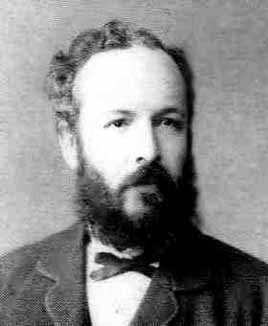Georg Ferdinand Ludwig Philipp Cantor (1845 - 1918) was a German mathematician. He is perhaps best known as the creator of set theory, which has become a fundamental theory in mathematics. Cantor established the importance of one-to-one correspondence between sets, defined infinite and well-ordered sets, and proved that the real numbers are "more numerous" than the natural numbers. (see Countable sets and Uncountable sets). In fact, Cantor's theorem implies the existence of an "infinity of infinities". He defined the cardinal and ordinal numbers,and their arithmetic.

Mathematicians call Infinite numbers – Transfinite numbers (well they would, wouldn’t they?)

Cantor formulated the Continuum Hypothesis.

There is a podcast by Marcus du Sautoy, accessible from this page:

One of the Famous People on this site.